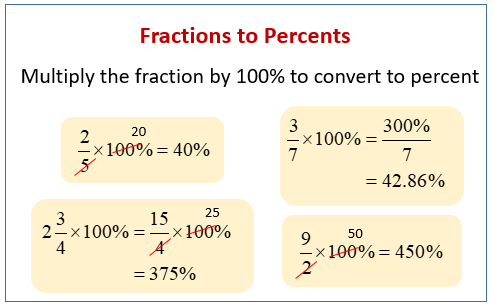# Know How to Convert Fractions to Percent and How Fractions are Different From Rational Numbers

When comparing quantities, we usually utilise fractions and percentages. Percentage or percent refers to fractions of a whole, whereas percent relates to how much of the whole thing is present and is easier to remember than a fraction. Therefore, one must know how to convert a given fraction to percent for one’s ease of understanding.

Furthermore, rational numbers are an important notion related to fractions. A rational number is a sort of real number that has the formula p/q where q is not equal to zero. A rational number is any fraction that has a non-zero denominator.

1/2, 1/5, 3/4, and other like numbers are examples of rational numbers.

The number “0” is also a rational number since it may be represented in a variety of ways, including 0/1, 0/2, 0/3, and so on. However, 1/0, 2/0, 3/0, and so on are not rational because they give us unlimited values.

Let’s learn about fractions, their conversion to percent and the Difference Between Fraction and Rational Numbers in detail.

## What is a Fraction?

A fraction is a number of equal parts or a portion of a total quantity. In other words, it reflects how many parts of a specific size were split by the total amount. A simple fraction of 1/2 has a numerator and a denominator. The denominator is written below the line, whereas the numerator is written above it.

The numerator represents some equal portions of a whole, whereas the denominator reflects the number of pieces that make up a whole, which cannot be 0. Think about the fraction 3/4. In this case, the numerator is 3 (three equal parts) and the denominator is 4 (four parts constitute a whole).

## What is Percent?

The term percent refers to a ratio or a quantity stated as a fraction of 100. The percentage symbol (%) is used to denote it. Here’s an example to help you understand how the percent reflects a fraction of 100. In fractional form, 35% is expressed as 35/100. In class, 50% of the students were male, which indicates that 50 students out of every 100 were male.

## How to Convert Fraction to Percent?

Simply multiply a fraction by 100 and divide the result by 100 to convert it to a percentage. The following examples will show you how to change a fraction into a percentage. The steps listed below will help you convert a fraction into a percentage:

• Convert the fraction into decimal form.
• To get a percent value, multiply the resulting decimal number by 100.

For Example,

Convert 3/5 to a percent.

Solution:

Step 1: Convert the given fraction 3/4 into decimal form

Step 2:  3/5 = 0.6

Step 3: Multiply the decimal number by 100: 0.6  × 100 = 60%

## Percentage Formula

A percentage is a figure or ratio with a denominator of 100. The following is the percentage formula:

For example, if we wish to find 20% of 150, it can be achieved as:

(20/ 100) × 150 = 3000/ 100

= 30

Thus, 20% of 150 is 30.

## Difference Between Fraction and  Rational Numbers

The following table illustrates some significant distinctions between fractional and rational numbers:

Note: Both fractions and rational numbers are real numbers.